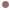Autres Articles de :
Discrete maps

1976 : The Hénon map

Christophe LETELLIER
25/11/2012The Hénon mapMichel Hénon

The Hénon map was discovered by Michel Hénon from the Nice Observatory in 1976 . This is a two-dimensional discrete map reading as

When it is plotted xn versus yn, this two-dimensional map obviously appears as a two-dimensional Logistic map as shown in Fig. 1 for the parameter values (a=1.4 and b=0.3) as originally used by Hénon.Fig. 1. Chaotic attractor solution to the Hénon map.A suspension of the Hénon map

Starting from the point of view that a discrete map can always be viewed as a first-return map to a Poincaré section of a flow, John Starrett & Craig Nicholas proposed a suspension of the Hénon map, that is, a three-dimensional flow whose first-return map to a Poincaré section is equivalent to the Hénon map . They used the orientation preserving map (quite similar to the original Hénon map)

with a=4/3 and b=-1 (Fig. 2).Fig. 2. Chaotic attractor solution to the orientation preserving Hénon map investigated by Starrett & Nicholas.

They then extracted three unstable periodic orbits from the chaotic attractor, constructed a suspension from these orbits using simple geometric transformations (a contraction, a bend, and a shift). They thus used the three-dimensional representation of these orbits to obtain a global model (using a projection on three multivariate polynomial functions) whose equations are provided in . A numerical simulation of the global model obtained and a computation of its first-return map to a Poincaré section are shown in Fig. 3. Compare the return map to the Hénon map they started from.Fig. 3. Suspension of the orientation preserving Hénon map and a first-return map to its Poincaré section.

 M. Hénon, A two-dimensional mapping with a strange attractor, Communications in Mathematical Physics, 50, 69-77, 1976.

 J. Starrett & C. Nicholas, A suspension of the Hénon map by periodic orbits, Chaos, Solitons & Fractals, 45, 1486-1493, 2012.